# Texas Go Math Grade 5 Lesson 9.4 Answer Key Apply Formulas

Refer to our Texas Go Math Grade 5 Answer Key Pdf to score good marks in the exams.
Test yourself by practicing the problems from Texas Go Math Grade 5 Lesson 9.4 Answer Key Apply Formulas.

## Texas Go Math Grade 5 Lesson 9.4 Answer Key Apply Formulas

Unlock the Problem

Mei Lin builds a jewelry box. The length of the box is 8 inches,
the width is 4 inches, and the height is 6 inches. How much velvet
does she need to line the bottom of the box?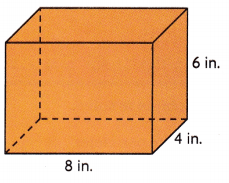STEP 1: Decide which formula to use.
The jewelry box is a rectangular prism. The base of the prism is a ___rectangle_______.
To find the amount of velvet needed to cover
the base of the prism, you need to find the ___area_______.
What formula should you use to find the area? _length X width_________

STEP 2: Find the area.
___a_______ = ___8_____ × _____4______
So, Mel Lin needs ____32______ square inches of velvet.

Remember
You have used these formulas
to find area, perimeter, and
volume.
A = l × w
P = 2l + 2w
V = l × w × h
Mel Lin needs 32 square inches of velvet  to cover
the base of the prism,

Explanation:
Given Mei Lin builds a jewelry box. The length of the box is 8 inches,
the width is 4 inches, and the height is 6 inches. To know how much velvet
does she need to line the bottom of the box  as the jewelry box is a rectangular prism.
The base of the prism is a rectangle  we need to find the area of rectangle,
we use the formula A = l X w = 8 inches X 4 inches = 32 square inches,
therefore Mel Lin needs 32 square inches of velvet  to cover the base of the prism.

Try This

Choose a formula to find the amount of space the jewelry box will occupy.
To find the amount of space, you need to find the __volume_________.
__v_____ = __l___ × ___w____ × __h_____ Write the formula.
___v____ = ___8____ × ___4____ × ___6____ Solve.
So, the jewelry box will occupy _____192______ cubic inches of space.
The jewelry box will occupy 192 cubic inches of space,

Explanation:
The amount of space the jewelry box will occupy as
the length of the box is 8 inches, the width is 4 inches and
the height is 6 inches so the volume =  length X width X height =
v = 8 inches X 4 inches X 6 inches = 192 cubic inches,
therefore the jewelry box will occupy 192 cubic inches of space.

Math Talk
Mathematical Processes

What if you only knew the area of the base and the height?
Explain how you could find the volume.
volume = area of the base X height,

Explanation:
If we knew the area of the base and the height then as the formula for
volume = area of the base X height.

Example

Find the unknown measurement.V = l × w × h
60 = ___5___ × __4____ × ☐
60 = _____20__ × ☐
Think: If I filled this prism with centimeter cubes, each layer would have 20 cubes.
How many layers of 20 cubes are equal to 60?
So, the unknown measurement is ___3_______ cm.
The unknown measurement is 3 cm,

Explanation:
Given volume of prism as 60, length of the prism as 5 cm, width as 4 cm and
unknown measurement is height of the prism, as we know
volume = length X width X height so 60 = 5 X 4 X h,
h= 60 ÷ 20 = 3 cm, therefore the unknown measurement is 3 cm.

Share and Show

Use a formula to solve.

Question 1.
Find the perimeter.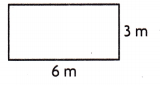P = _____18 m______
Perimeter is 18 m,

Explanation:
Given rectangle has length l is 6 m and width w is 3 m as
we know perimeter of rectangle is 2(l + w) so P = 2(6m + 3m),
P = 2(9 m) = 18 m.

Question 2.
Find the volume.V = ____343 cubic ft_______
The volume is 343 cubic ft,

Explanation:
Given cube has edge 7 ft as we know volume of cube =
edge X edge X edge = 7 ft X 7 ft X 7 ft = 343 cubic ft.

Question 3.
Find the unknown measurement.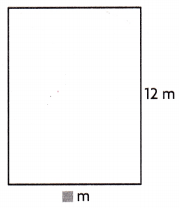A = 108 sq m ☐ = ____9____ m
The unknown measurement is 9 m,

Explanation:
Given area of rectangle as 108 sq m and width is 12 m,
let l be the unknown measurement as we know A = length X width, 108 = l X 12,
So l = 108 ÷ 12 = 9 m.

Math Talk
Mathematical Processes

Explain how you found the unknown measurement in Exercise 3.
Using the formula of area of rectangle,

Explanation:
Given is Area as A and the figure is a rectangle and even width is given,
so the unknown measurement is length using the formula of area of rectangle
a = l X w we can calculate the unknown measurement.

Problem Solving

Question 4.
H.O.T. The perimeter of a rectangle is 40 ft. The length is 15 ft.
Explain how you can find the width of the rectangle.
The width of the rectangle is 5 ft,

Explanation:
Given the perimeter of a rectangle is 40 ft the length is 15 ft,
and let w be the width of the rectangle as we know perimeter of
rectangle p = 2(l + w), So 40 = 2(15 + w),
40 = 30 + 2w, 2w = 10, w = 10 ÷ 2 = 5 ft,
therefore the width of the rectangle is 5 ft.

Question 5.
H.O.T. Multi-Step The area of a square is 64 sq cm. What is the perimeter of the square?
The perimeter of the square is 32 cm,

Explanation:
Given the area of as square is 64 sq cm as we know
area of square is side X side so the side of the square
s X s = 64 sq cm, s X s = 8 cm X 8 cm, therefore s = 8 cm,
as we know the perimeter of the square is 4 X side,
so p = 4 X 8 = 32 cm.

Problem Solving

Use the diagram for 6-8.Question 6.
Martita uses the diagram to the right to make a cedar chest.
She wants to add a copper border around of the base of the chest.
How much of the copper border should she buy?
The copper border Martita should  buy is (16 in X 10 in) = 160 square in,

Explanation:
Given Martita uses the diagram to the right to make a cedar chest.
She wants to add a copper border around of the base of the chest.
As base of the chest is in rectangular prism the formula is
area of the base = length X width, therefore the copper border
should she buy is (16 in X 10 in) = 160 square in.

Question 7.
Multi-Step Martita wants to line the bottom of the chest with felt.
She will use two colors of felt so that each color covers half of the area.
How much felt does she need for each color?
Felt Martita need for each color is 26 in,

Explanation:
As Martita wants to line the bottom of the chest with felt.
She has used two colors of felt so that each color covers half of the area.
So felt does she need as we have perimeter of the base as 2l + 2w upon
substituting (2 X 16 in+ 2 X 10 in) = (32 + 20) = 52 in.

Question 8.
H.O.T. The instructions for making the chest are smudged.
Martita cannot read what the height of the chest should be.
She knows that the volume should be 6,400 cubic inches.
Explain how you can find the height of the chest.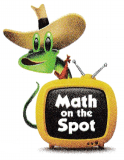The chest should be 40 in,

Explanation:
As the instructions for making the chest are smudged.
and Martita cannot read what the height of the chest should be,
She knows that the volume should be 6,400 cubic inches and
length is 16 in, width is 10 in as we know Volume = length X width x height so
6400 cubic inches = 16 in X 10 in X h,
h = 6400 cubic inch ÷ 160 square in,
h = 40 in.

Question 9.
A thin plastic cover must be placed on top of this box. How much plastic is needed?(A) 525 sq cm
(B) 1,400 sq cm
(C) 21,000 sq cm
(D) 600 sq cm
Plastic needed is (B) 1,400 sq cm,

Explanation:
Given to find a thin plastic cover must be placed on top of the above  box,
As base and top same we have base = length X width,
So plastic needed is only 40 cm X 35 cm = 1,400 sq cm which matches with (B).

Question 10.
Representations Emily wants to put a rosebud wallpaper border on
the walls of her room. The border will form a rectangle around the room.
Which formula should she use to find the length of wallpaper she needs?
(A) V = l × w × h
(B) A = l × w
(C) P = 4s
(D) P = l + l + w + w
To find the length of wallpaper Emily needs formula of  P = l + l + w + w,
(D),

Explanation:
Given Emily wants to put a rosebud wallpaper border on the walls of her room.
The border will form a rectangle around the room.
So formula should she use to find the length of wallpaper she needs means
perimeter(means border) from the formula of the perimeter she can find the
length as we know perimeter of rectangle is P= 2(l + w) or P = l + l + w + w
which matches with (D).

Question 11.
Multi-Step Spencer and Kamden are comparing the size of their suitcases.
Both suitcases are shaped like rectangular prisms. Spencer’s suitcase is
15 inches long, 18 inches wide, and 5 inches tall. Kamden’s suitcase is
14 inches long, 15 inches wide, and 7 inches tall. Which statement is correct?
(A) Kamden’s suitcase can hold more because it has a greater volume.
(B) Kamden’s suitcase can hold more because it has a greater area.
(C) Spencer’s suitcase can hold more because it has a greater volume.
(D) Spencer’s suitcase can hold more because it has a greater area.
Both (A) Kamden’s suitcase can hold more because it has a greater volume,
(D) Spencer’s suitcase can hold more because it has a greater area,
So (A) and (D)statements are correct,

Explanation:
As we know Rectangular prism formula as Volume = l X w X h and
surface area A = 2(lw + lh + wh), so first we calculate volume and area of
Spencer’s suitcase of 15 inches long, 18 inches wide, and 5 inches tall
V = 15 X 18 X 5 = 1,350 cubic inches and Area = 2(15 X 18 + 15 X  5 + 18  X  5),
A = 2 X (270 + 75 + 90), A = 2 X 435, A = 870 square inches,
Now we calculate volume and area of Kamden’s suitcase of
14 inches long, 15 inches wide, and 7 inches tall V = 14  X  15 X  7 = 1,470 cubic inches,
A = 2(14 x 15 + 14 X 7 + 15 X 7), A = 2 (210 + 98 + 105),
A = 2 X 413, A = 826 square inches, Now comparing between areas and volumes of
Kamden’s suitcase can hold more because it has a greater volume and
Spencer’s suitcase can hold more because it has a greater area,
So (A) and (D)statements are correct.

Texas Test Prep

Question 12.
Julio’s room has a rectangular floor. He covers the floor with 180 square feet of carpet.
If the width of the room is 9 feet, what is the length of the room?
(A) 2 feet
(B) 20 feet
(C) 58 feet
(D) 20 square feet
(B) 20 feet,

Explanation:
Given Julio’s room has a rectangular floor.
He covers the floor with 180 square feet of carpet.
If the width of the room is 9 feet,  So the length of the room as
Area of floor= length X width,
180 square feet = l X 9 feet,
l= 180 square feet ÷ 9 = 20 feet which matches with (B).

### Texas Go Math Grade 5 Lesson 9.4 Homework and Practice Answer Key

Use a formula to solve.

Question 1.
Find the perimeter.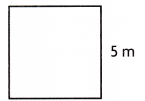P = ____20 m________
Perimeter of given square is 20 m,

Explanation:
Given side of the square as 5m as perimeter of square is
4 X side, therefore P = 4 x 5m = 20 m.

Question 2.
Find the volume.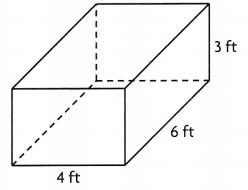V = ____72 cubic ft________
Volume is 72 cubic ft,

Explanation:
Given to find the volume of  rectangular cube of
length 4 ft, width 6 ft and height 3 ft,
As formula for volume =  length X width X height =
4 ft X 6 ft X 3 ft = 72 cubic ft.

Find the unknown measurement.

Question 3.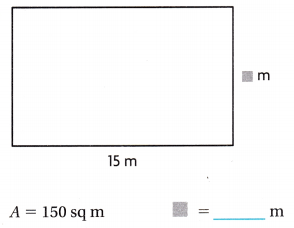Unknown measurement is 10 m,

Explanation:
Given area of rectangle as 150 sq m and length as 15 m,
so unknown measurement is width w as we know area of rectangle is
A = length X width,
150 sq m = 15 m X w,
w = 150 sq m ÷ 15 m = 10 m.

Question 4.Unknown measurement is 14 in,

Explanation:
Given perimeter of rectangle as 42 in and length as 7 in,
so unknown measurement is width w as we know perimeter of rectangle is
P = 2(length + width),
42 in = 2(7in + w),
42 in = 14 in + 2w,
2w = 42 in – 14 in = 28 in
so w = 28 in ÷ 2 in = 14 in,
therefore unknown measurement is 14 in.

Problem Solving

Question 5.
Yuan is mailing the package shown at right. He needs to find the volume of
the package so he can decide what size bag of packing materials to buy
to fill the package. What is the volume of the package?The volume of the package is 6,912 cubic cm,

Explanation:
Given Yuan is mailing the package shown at right. He needs to find the volume of
the package so he can decide what size bag of packing materials to buy
to fill the package as it has length 18 cm width 16 cm and height 24 cm,
As volume = length X width X height,
V = 18 cm X 16 cm X 24 cm,
V = 6,912 cubic cm, therefore the volume of the package is 6,912 cubic cm.

Lesson Check

Question 6.
A classroom floor will be covered with carpet. Which formula is needed
to find the amount of carpet needed?
(A) P = 2l + 2w
(B) V = l × w × h
(C) A = l × w
(D) P = 4s
Amount of carpet needed is A = l × w, (C),

Explanation:
Given a classroom floor will be covered with carpet. Formula is needed
to find the amount of carpet needed will be base area so as we know
Base Area A = length X width, A = l x w which matches with (C).

Question 7.
A storage locker measures 5 feet by 6 feet by 14 feet.
How much space in the warehouse will the storage locker occupy?
(A) 420 cubic feet
(B) 154 cubic feet
(C) 1,176 cubic feet
(D) 120 cubic feet
(A) 420 cubic feet,

Explanation:
Given a storage locker measures 5 feet by 6 feet by 14 feet.
So space in the warehouse will the storage locker occupy is
5 feet X 6 feet X 14 feet = 420 cubic feet which matches with (A).

Question 8.
The diagram below shows the number of square tiles on a playhouse floor.
The length and width of one tile are labeled in inches.What is the total area of the playhouse floor?
(A) 144 square inches
(B) 2,304 square inches
(C) 2,880 square inches
(D) 216 square inches
(C) 2,880 square inches,

Explanation:
Given the diagram that shows the number of square tiles on a playhouse floor.
The length and width of one tile are labeled in inches.
Total length is 12 in X 5 tiles = 60 in,
Total width is 12 in X 4 = 48 in, therefore total area of the
playhouse floor is length X width = 60 in X 48 in = 2,880 square inches,
which matches with (C).

Question 9.
Multi-Step At Hal’s Shipping Store, the cost of mailing a box increases
as the size of the box increases. Ming mails a box that measures
11 inches by 12 inches by 9 inches. Eli mails a box that measures
12 inches by 14 inches by 6 inches. Which statement is correct?
(A) Ming’s cost is less because her box has less volume.
(B) Ming’s and Eli’s costs are the same because the boxes have the same volume.
(C) Eli’s cost is greater because his box has greater volume.
(D) Ming’s cost is greater because her box has greater volume.
(D) Ming’s cost is greater because her box has greater volume,

Explanation:
Given at Hal’s Shipping Store, the cost of mailing a box increases
as the size of the box increases. Ming mails a box that measures
11 inches by 12 inches by 9 inches. Eli mails a box that measures
12 inches by 14 inches by 6 inches.
As we know Volume = l X w X h first we calculate volume of
Ming mails box that measures 11 inches by 12 inches by 9 inches.
V = 11 X 12 X 9 = 1,188 cubic inches
Now we calculate volume of Eli mails box that measures
12 inches by 14 inches by 6 inches
V = 12  X 14 X  6 = 1,008 cubic inches,
Now comparing the volumes Ming mails box measures greater so
Ming’s cost is greater therefore statement (D) Ming’s cost is greater
because her box has greater volume is correct.

Question 10.
Multi-Step Angela is lining the bottom of some cabinet drawers with paper.
How much paper will she need to cover the bottom of three drawers?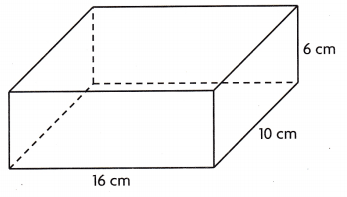(A) 960 sq cm
(B) 480 sq cm
(C) 160 sq cm
(D) 2,880 sq cm